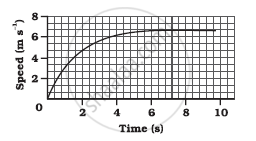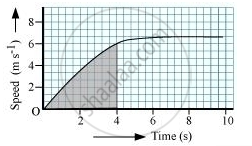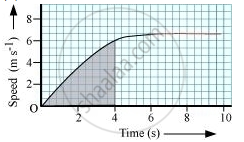Share

# The Speed-time Graph for a Car is Shown in the Following Figure - CBSE Class 9 - Science

ConceptGraphical Representation of Motion - Distance-time Graphs

#### Question

The speed-time graph for a car is shown in the following figure(a) Find out how far the car travels in the first 4 seconds. Shade the area on the graph that represents the distance travelled by the car during the period.

(b) Which part of the graph represents uniform motion of the car?

#### Solution

(a)The shaded area which is equal to 1/2xx4xx6=12m represents the distance travelled by the car in the first 4 s.

(b)The part of the graph in red colour between time 6 s to 10 s represents uniform motion of the car.

Is there an error in this question or solution?

#### Video TutorialsVIEW ALL 

Solution The Speed-time Graph for a Car is Shown in the Following Figure Concept: Graphical Representation of Motion - Distance-time Graphs.
S